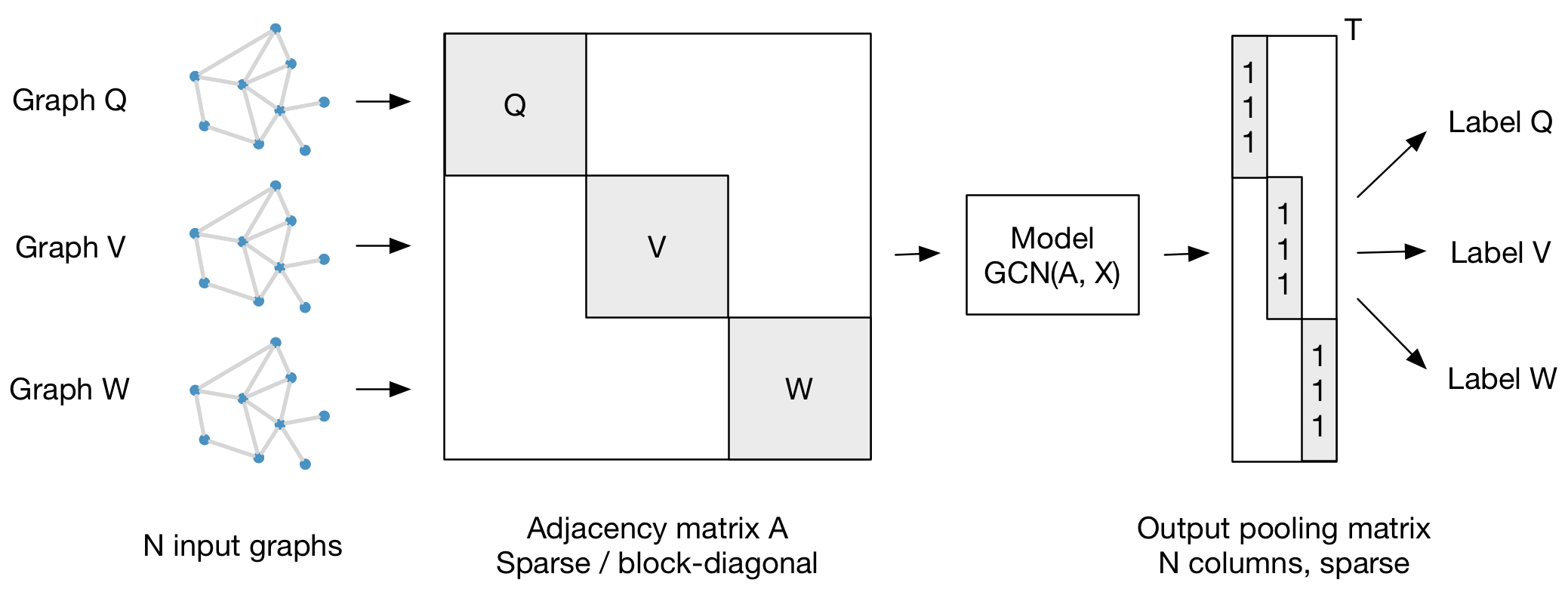# Graph Convolutional Networks

This is a TensorFlow implementation of Graph Convolutional Networks for the task of (semi-supervised) classification of nodes in a graph, as described in our paper:

Thomas N. Kipf, Max Welling, Semi-Supervised Classification with Graph Convolutional Networks (ICLR 2017)

For a high-level explanation, have a look at our blog post:

Thomas Kipf, Graph Convolutional Networks (2016)

## Installation

``````python setup.py install
``````

## Requirements

• tensorflow (>0.12)
• networkx

## Run the demo

``````cd gcn
python train.py
``````

## Data

In order to use your own data, you have to provide

• an N by N adjacency matrix (N is the number of nodes),
• an N by D feature matrix (D is the number of features per node), and
• an N by E binary label matrix (E is the number of classes).

Have a look at the `load_data()` function in `utils.py` for an example.

In this example, we load citation network data (Cora, Citeseer or Pubmed). The original datasets can be found here: http://www.cs.umd.edu/~sen/lbc-proj/LBC.html. In our version (see `data` folder) we use dataset splits provided by https://awesomeopensource.com/project/kimiyoung/planetoid (Zhilin Yang, William W. Cohen, Ruslan Salakhutdinov, Revisiting Semi-Supervised Learning with Graph Embeddings, ICML 2016).

You can specify a dataset as follows:

``````python train.py --dataset citeseer
``````

(or by editing `train.py`)

## Models

You can choose between the following models:

## Graph classification

Our framework also supports batch-wise classification of multiple graph instances (of potentially different size) with an adjacency matrix each. It is best to concatenate respective feature matrices and build a (sparse) block-diagonal matrix where each block corresponds to the adjacency matrix of one graph instance. For pooling (in case of graph-level outputs as opposed to node-level outputs) it is best to specify a simple pooling matrix that collects features from their respective graph instances, as illustrated below:## Cite

Please cite our paper if you use this code in your own work:

``````@inproceedings{kipf2017semi,
title={Semi-Supervised Classification with Graph Convolutional Networks},
author={Kipf, Thomas N. and Welling, Max},
booktitle={International Conference on Learning Representations (ICLR)},
year={2017}
}
``````

Get A Weekly Email With Trending Projects For These Topics
No Spam. Unsubscribe easily at any time.
Python (838,789
Network (37,567
Graph (24,405
Convolutional Neural Networks (12,631
Matrix (10,860